Find x + y + z + w in the given figure.

# Find in the given figure.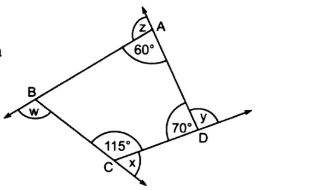1. A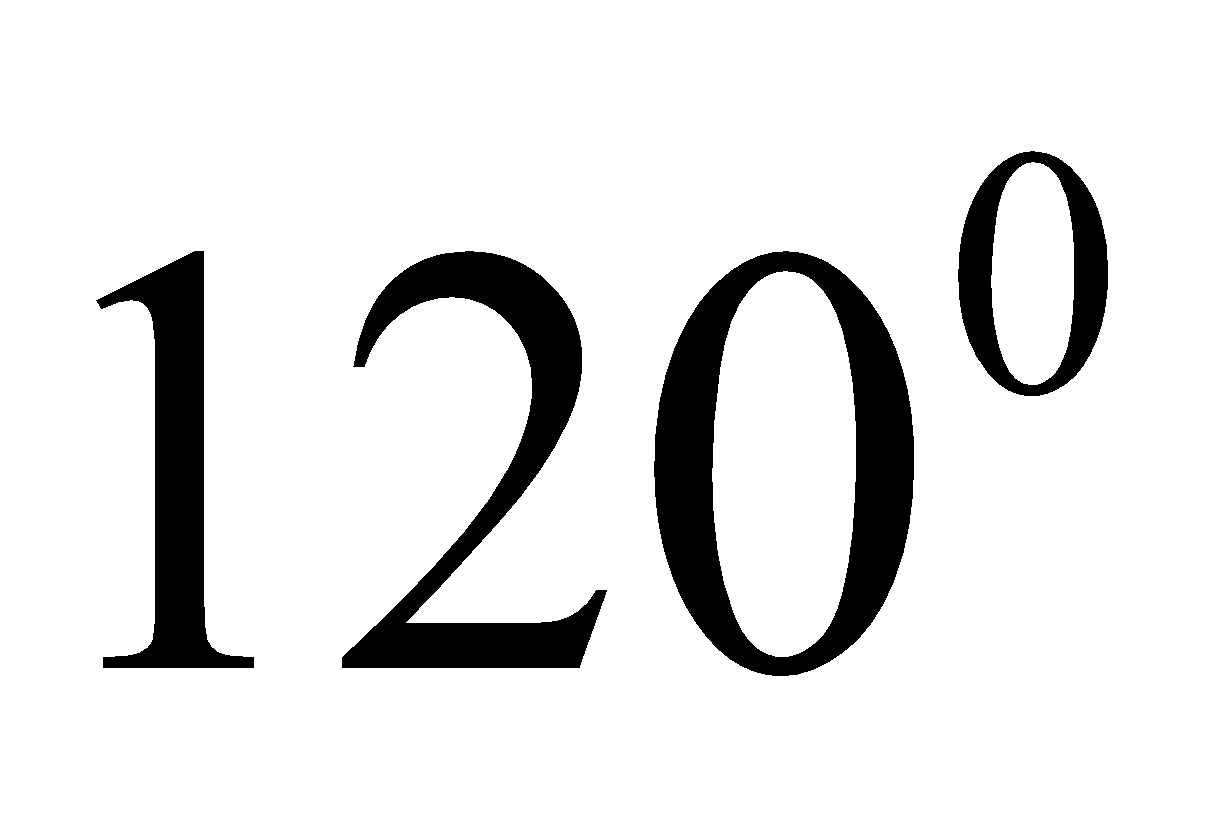2. B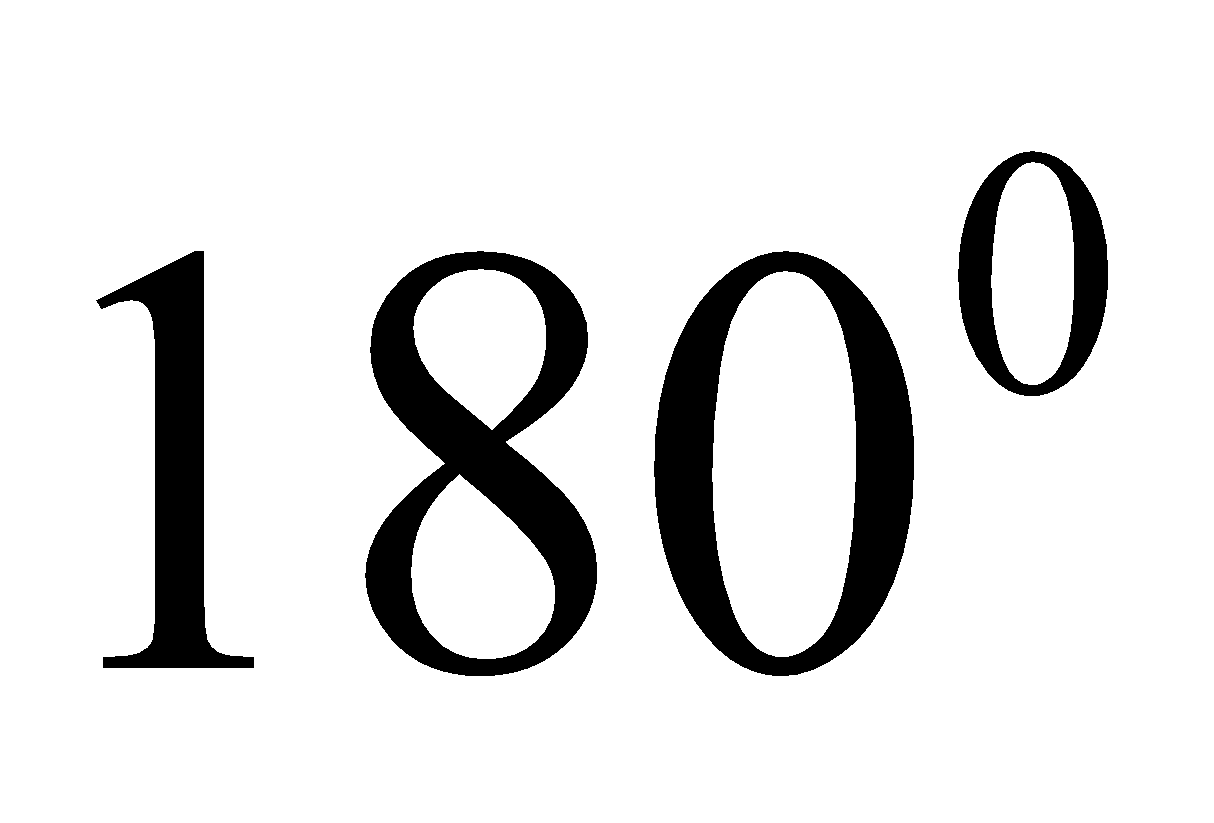3. C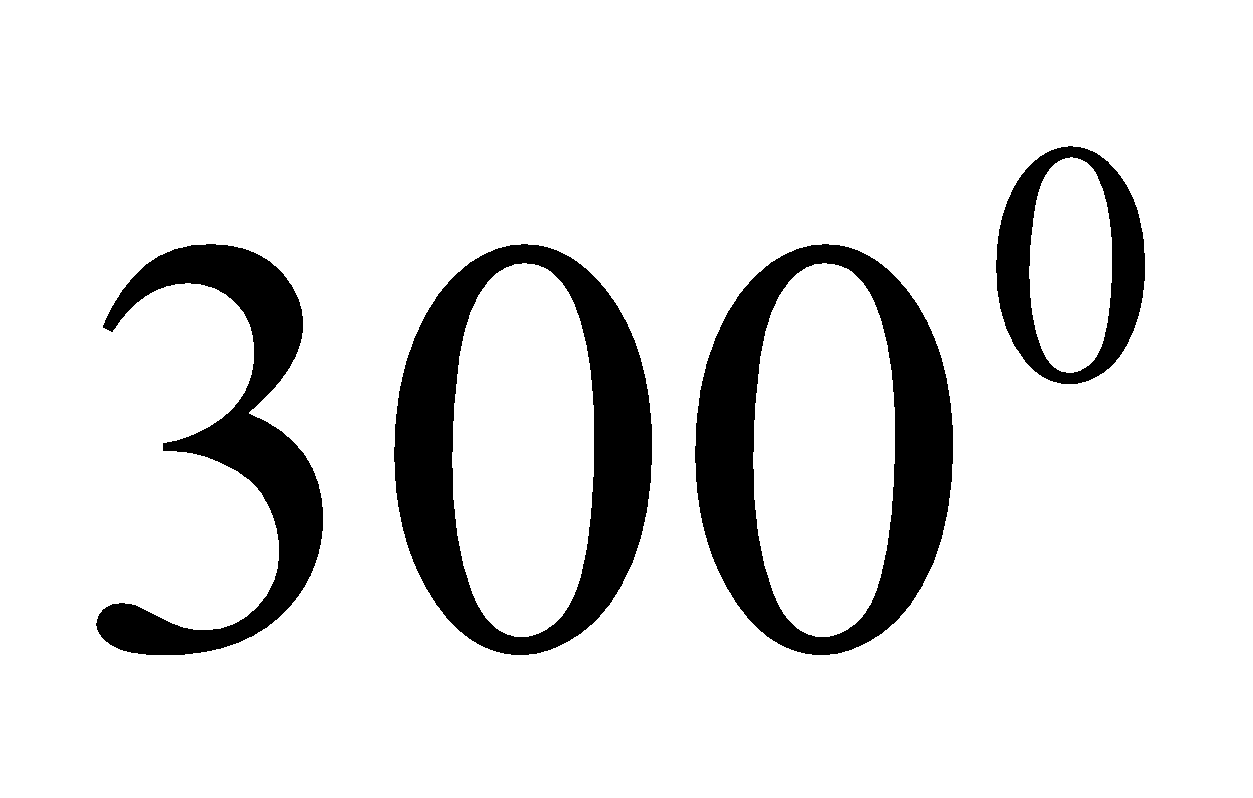4. D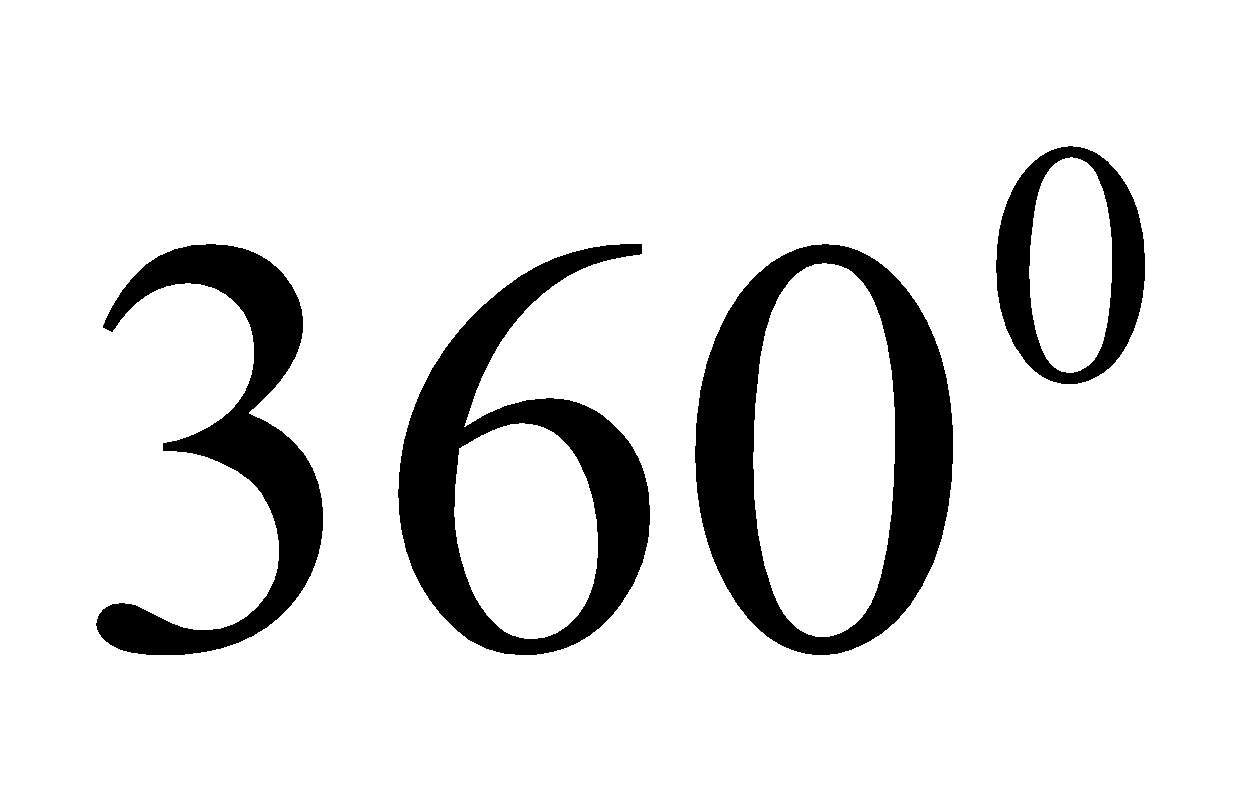Fill Out the Form for Expert Academic Guidance!l

+91

Live ClassesBooksTest SeriesSelf Learning

Verify OTP Code (required)

### Solution:

Concept- Here we will proceed by using the sum of interior angle properties of the quadrilateral because the given shape is quadrilateral. Also, we will use exterior angle property.
Since the sum of the measures of interior angles of a quadrilateral isAlso,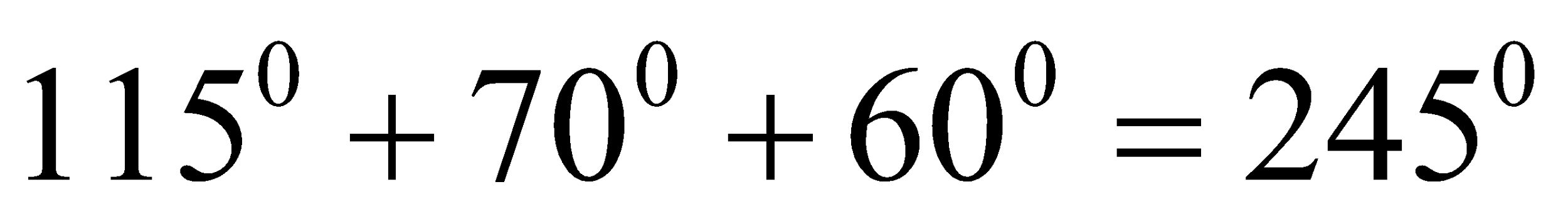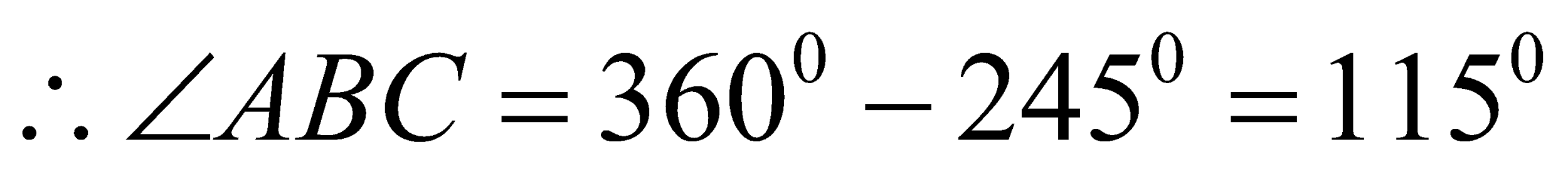Now,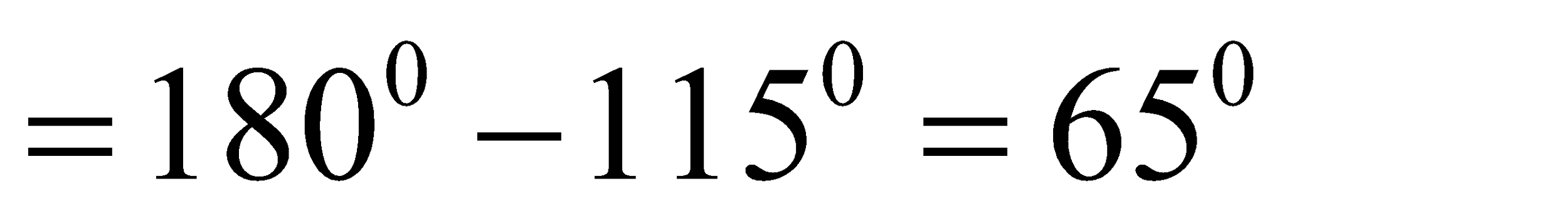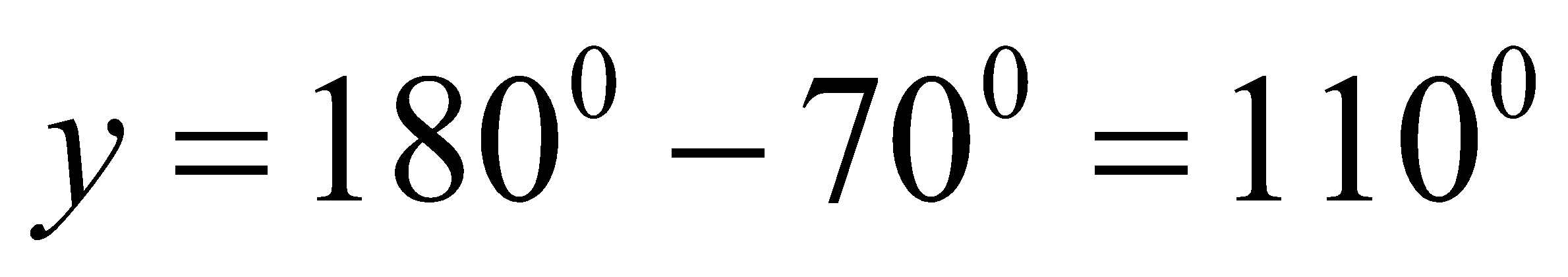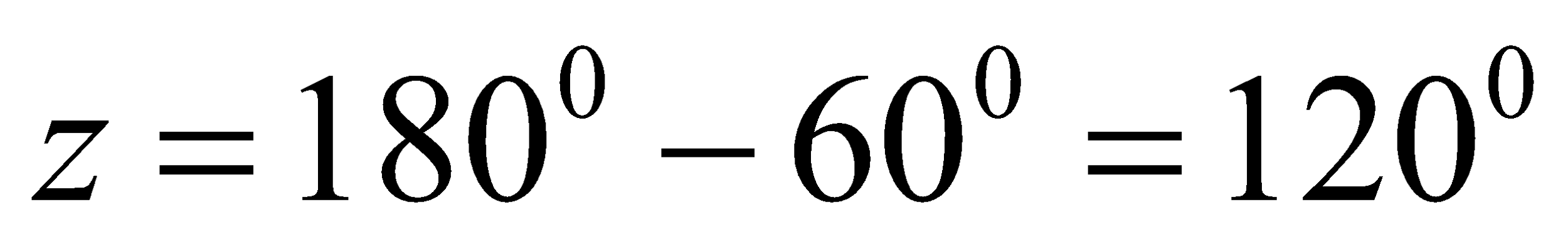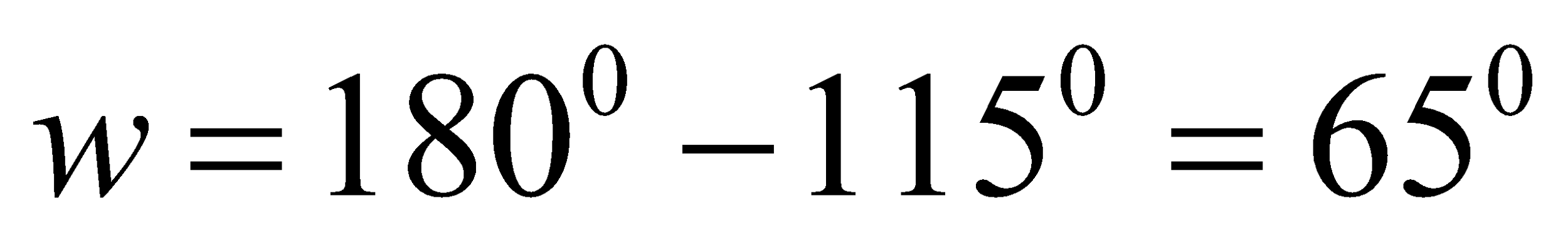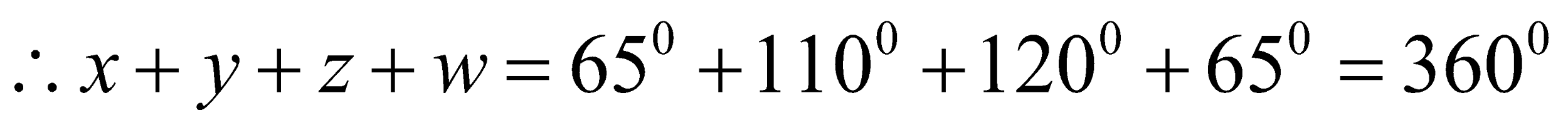Hence,the answer is option 4.

## Related content

 Area of Square Area of Isosceles Triangle Pythagoras Theorem Triangle Formula Perimeter of Triangle Formula Area Formulae Volume of Cone Formula Matrices and Determinants_mathematics Critical Points Solved Examples Type of relations_mathematics+91

Live ClassesBooksTest SeriesSelf Learning

Verify OTP Code (required)